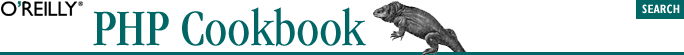home | O'Reilly's CD bookshelfs | FreeBSD | Linux | Cisco | Cisco ExamSymbols & Numbers | A | B | C | D | E | F | G | H | I | J | K | L | M | N | O | P | Q | R | S | T | U | V | W | X | Y | Z

Index: K

kerning words and letters: 15.5.3. Discussion
keys, array
associative vs. numeric arrays: 4.1. Introduction
checking arrays for specific: 4.11.1. Problem
merged arrays and: 4.8.3. Discussion
numeric: 4.7.3. Discussion
negative numbers in: 4.2.3. Discussion
storing multiple elements in single key: 4.3.1. Problem
strings as: 4.1. Introduction
6.9.3. Discussion
uniqueness of: 4.3.3. Discussion
keys, encryption (see encryption)
key/value pairs, array
preserving association between in array sorting: 4.17.3. Discussion
relationship between, preserving in sorts: 4.18.3. Discussion
specifying with => operator: 4.1. Introduction
swapping keys and values with array_flip( ): 4.12.3. Discussion
key/value pairs for DBM databases: 10.3.3. Discussion
keywords
class: 7.1. Introduction
extends: 7.1. Introduction
function: 6.1. Introduction
7.1. Introduction
new: 7.1. Introduction
instantiating objects: 7.2.2. Solution
parent::: 7.8.3. Discussion
in PHPDoc comment blocks: 21.9.3. Discussion
var: 7.1. Introduction

Symbols & Numbers | A | B | C | D | E | F | G | H | I | J | K | L | M | N | O | P | Q | R | S | T | U | V | W | X | Y | Z